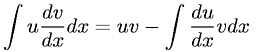Calculus > Integration/IntegralsShowing results 1 to 9 of 9, on page 1 of 1
 DESCRIPTION EQUATION Fundamental Theorem of Integrals of Derivatives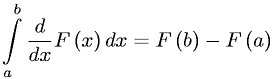Integral of cosecant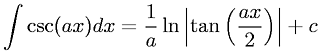Integral of cosineIntegral of powers not equal to -1Integral of reciprocal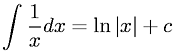Integral of secant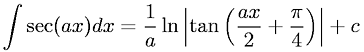Integral of sine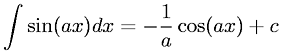Integral of tangent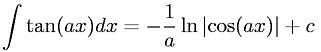Integration by parts